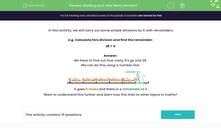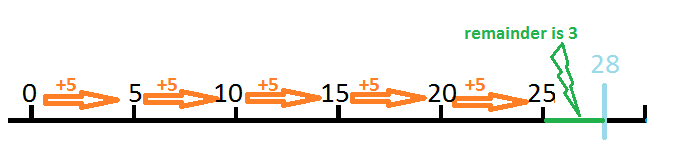# Dividing by 5: How Many Remain?

In this worksheet, students divide two-digit numbers by 5 and note down any remainders.Key stage:  KS 2

Curriculum topic:   Number: Multiplication and Division

Curriculum subtopic:   Multiply/Divide Formally

Difficulty level:#### Worksheet Overview

In this activity, we will carry out some simple divisions by 5 with remainders.

e.g. Calculate this division and find the remainder:

28 ÷ 5

We have to find out how many 5's go into 28.

We can do this using a number line.It goes 5 times and there is a remainder of 3.

Want to understand this further and learn how this links to other topics in maths?

### What is EdPlace?

We're your National Curriculum aligned online education content provider helping each child succeed in English, maths and science from year 1 to GCSE. With an EdPlace account you’ll be able to track and measure progress, helping each child achieve their best. We build confidence and attainment by personalising each child’s learning at a level that suits them.

Get started